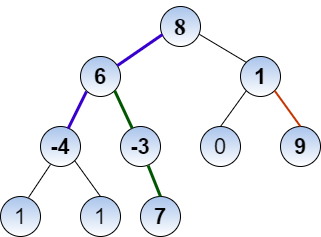New update is available. Click here to update.

# Path Sum lll

Last Updated: 11 Feb, 2021
Difficulty: Hard

## PROBLEM STATEMENT

#### You have been given a labelled binary tree having integer value (except zero) corresponding to all its nodes. You have to find the number of possible ways to make the sum of nodes (in a particular path) exactly equal to ‘k’.

##### Note:
``````i) Nodes considered for the sum should be from parent to child.
ii) For a particular way, you can only consider nodes having the common path.
iii) Two ways are different from each other if they have an unequal number of nodes (for making sum equal to k) or at least one node is different between both the ways.
``````
##### For example :``````For the binary tree shown in the figure, the possible ways/paths are [8, 6, -4], [6, -3, 7], and [1, 9] for a sum of nodes equal to 10.
``````
##### Input Format:
``````The first line contains an integer 'T' which denotes the number of test cases to be run. Then the test cases are as follows.

The first line of each test case contains an integer ‘K’ denoting the required sum.

The second line of each test case contains elements of the tree in the level order form. The line consists of values of nodes separated by a single space. In case a node is null, we take -1 in its place.

For example, the input for the tree depicted in the below image would be :
````````````1
2 3
4 -1 5 6
-1 7 -1 -1 -1 -1
-1 -1
``````

#### Explanation :

``````Level 1 :
The root node of the tree is 1

Level 2 :
Left child of 1 = 2
Right child of 1 = 3

Level 3 :
Left child of 2 = 4
Right child of 2 = null (-1)
Left child of 3 = 5
Right child of 3 = 6

Level 4 :
Left child of 4 = null (-1)
Right child of 4 = 7
Left child of 5 = null (-1)
Right child of 5 = null (-1)
Left child of 6 = null (-1)
Right child of 6 = null (-1)

Level 5 :
Left child of 7 = null (-1)
Right child of 7 = null (-1)

The first not-null node(of the previous level) is treated as the parent of the first two nodes of the current level. The second not-null node (of the previous level) is treated as the parent node for the next two nodes of the current level and so on.
The input ends when all nodes at the last level are null(-1).
``````
##### Note :
``````The above format was just to provide clarity on how the input is formed for a given tree.
The sequence will be put together in a single line separated by a single space. Hence, for the above-depicted tree, the input will be given as:

1 2 3 4 -1 5 6 -1 7 -1 -1 -1 -1 -1 -1
``````
##### Output Format:
``````For each test case, print the number of ways to make the sum of nodes equal to k under the given conditions.
``````
##### Note:
``````You do not need to print anything, it has already been taken care of. Just implement the given function.
``````
##### Constraints:
``````1 <= T <= 10
0 <= N <= 500
-10^9 <= K <= 10^9
-100 <= data <= 100

Where ‘T’ is the number of test cases, and ‘N’ is the total number of nodes in the binary tree, ‘K’ is the required sum, and “data” is the value of the binary tree node. “data”  equal to -1 represents a NULL value.

Time Limit: 1sec
``````## Approach 1

The basic idea of this approach is to compute the sum from leaf to root node by applying pre-order traversal on the tree.

First, you have to declare a vector which will store nodes in a particular path. Let’s assume its name is “path”. You have to also declare a variable (Let’s assume the name “ans”) to store the answer (number of ways). Initialize “ans” with 0.

Now consider a recursive function which will be used to calculate the number of ways for the given parameters:

``void helper(TreeNode *root, vector<int> &path, int K) ``

Where “root” denotes the root node of the constructed binary tree. “path” and ‘K’ denotes the vector for storing a particular path and required sum, respectively.

1. Check, if root = NULL, returns.
2. Traverse the tree in a preorder manner and store all the nodes in the vector named “path”. This is done as:
• path.push_back(root->data)
• helper(root->left, path, K)
• helper(root->right, path, K)
3. At each recursive call also check if there is a path with sum as K or not. This is done as:
• Count = 0
• For i from path.size()-1 to 0
• Count += path[i]
• If count = K => ans = ans + 1
4. After this, remove the current node from the path, so as to take other unique paths. path.pop_back()
5. Return the value of ans.